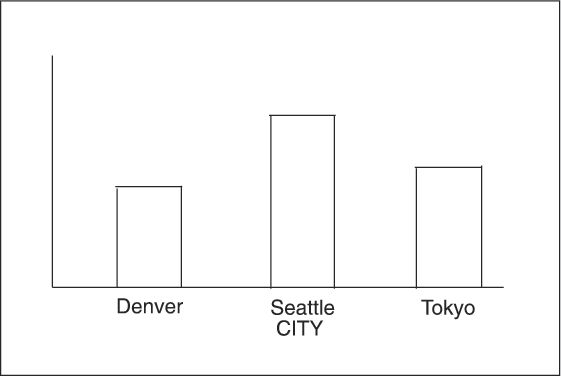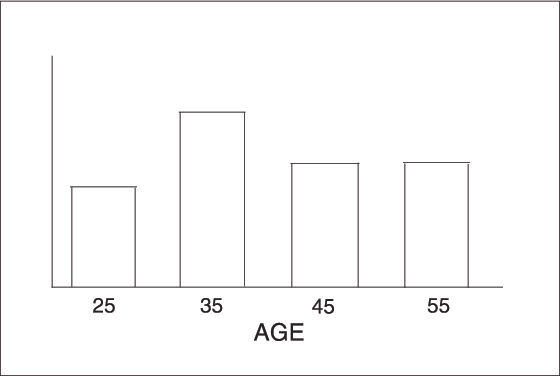# GBARLINE Procedure

## Definition: Midpoints

Midpoints are the values of the chart variable that identify categories of data. By default, midpoints are selected or calculated by the procedure. The way the procedure handles the midpoints depends on whether the values of the chart variable are character, discrete numeric, or continuous numeric.

## Character Values

A character chart variable generates a midpoint for each unique value of the variable. In the following example, the chart variable CITY contains the names of three different cities, and each city is a midpoint, resulting in three midpoints for the chart:
Character MidpointsBy default, character midpoints are arranged in alphabetic order. If a character variable has an associated format, then the values are arranged in order of the formatted values.

## Continuous Numeric Values

A continuous numeric variable generates midpoints that represent ranges of values. By default, the GBARLINE procedure determines the number of uniform ranges (LEVELS), calculates the number of observations in each range, and then computes the TYPE= statistic based on this frequency. A value that falls exactly on a range boundary is placed in the higher range.
In the following example, the numeric variable AGE has been divided into five equal levels that span the data range. The horizontal axis tick values are at the midpoint of each level.
Continuous Numeric MidpointsBy default, midpoints of ranges are arranged in ascending order.

## Selecting and Ordering Midpoints

For character or discrete numeric values, you can use the MIDPOINTS= option to rearrange the midpoints or to exclude midpoints from the chart. For example, to change the default alphabetic order of the midpoints in Character Midpoints, specify the following midpoints:
`midpoints="Tokyo" "Denver" "Seattle"`
To exclude the midpoint for Denver, specify the following midpoints:
`midpoints="Tokyo" "Seattle"`
In this case, values excluded by the option are not included in the calculation of the chart statistic.
You can order or select discrete numeric midpoint values just as you do character values, but you omit the quotation marks when specifying numeric values.
For continuous numeric variables, use the LEVELS= or MIDPOINTS= option to change the number of midpoints. These options can also control the range of values each midpoint represents or change the order of the midpoints. To control the range of values each midpoint represents, use the MIDPOINTS= option to specify the midpoint value of each range. For example, to select the ranges 20–29, 30–39, and 40–49, specify the following values:
`midpoints=25 35 45;`
Alternatively, to select the number of midpoints that you want and let the procedure calculate the ranges and midpoints, use the LEVELS= option.
You can also use formats to control the ranges of continuous numeric variables, but in that case the values are no longer continuous but become discrete.
Note: You cannot use the MIDPOINTS= option to exclude continuous numeric values from the chart. This is because values below or above the ranges specified by the option are automatically included in the first and last midpoints. To exclude continuous numeric values from a chart, use a WHERE statement in a DATA step or the WHERE= data set option.
See also the descriptions of the options LEVELS=number-of-midpoints | ALL and